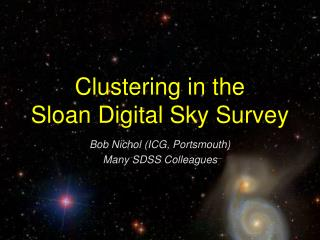DownloadDownload PresentationClustering in the Sloan Digital Sky Survey

# Clustering in the Sloan Digital Sky Survey

Télécharger la présentation## Clustering in the Sloan Digital Sky Survey

- - - - - - - - - - - - - - - - - - - - - - - - - - - E N D - - - - - - - - - - - - - - - - - - - - - - - - - - -
##### Presentation Transcript

1. Clustering in the Sloan Digital Sky Survey Bob Nichol (ICG, Portsmouth) Many SDSS Colleagues

2. Outline • Statistics of clustering • SDSS overview and update • What have we learnt from p(k)? • Caution about fair samples • Features in p(k)? • The Future

3. Clustering in the Universe Measuring the distribution of matter in the Universe is a fundamental goal of physics as it tests theories of gravity and the creation of mass in the Universe dV r or dV Alternatively, can express the correlation function in terms of the power spectrum of density fluctuations

4. On large scales, we expect fluctuation to be small so perturbation theory can be applied. On small scales, the density field will be highly evolved and non-linear (see CMBfast) ADD DEFINITION OF Tk and primodial P(k) Primodial P(k) The “break” gives mh2 due to horizon at matter-radiation equality k / mh2 Mpc-1

5. P(k) and cosmology • Baryon fraction via suppression of power on small-scales and oscillations (the non-existence of strong oscillations rules out a pure baryon universe) • Large scales tells us the primodial power spectrum (coming out of inflation) • Neutrinos cause suppression on small-scales as well (degenerate with baryons) • Total mass density of Universe from “break” (mh2 Therefore, like the CMB power spectrum, precision measurements of P(k) provide important information on the contents of the Universe • However, we see galaxies which are not a continuous tracer of the mass and are biased: • Galaxy evolution is messy gas physics and thus hard to predict from first principles (biasing must be empirically determined)

6. SDSS: Precision Measurement of P(k) - photometry & completeness DR2: 367,000 spectra, 3324 sq degs (half-way) Done 07/2005: ~700,000 spectra, 8000 sq degs Extension (2005-2007): Legacy, SNe, Galaxy

7. 205,000 redshifts, 2500 sq degs (sample12)

8. Fair Sample? The inclusion of the “SDSS Great Wall” (Gott et all. 2003) has a 40% effect on P(k)

9. SDSS P(k) of Tegmark et al. mh = 0.213 (11% error), assuming h=0.72 and spatially flat Combine with CMB data (spatially flat), then we require Dark Energy to fill the Universe!

10. Large Scale P(k)Have we been the beyond the “break”? Hard as fluctuation are small (linear regime) demanding homogeneous photometry and selection of galaxies over large volumes Luminous Red Galaxies (Massive Ellipticals) maybe the answer

11. ubiquous (nP~1) • passively evolving & luminous • Biased (but scale-dependent? Potential to model it)

12. Using hydro sims, for k < (1 Mpc)-1, the shape of Pflux(k) matches primordial P(k) (see Croft et al. 1998) P(k,z) At higher z, the effect of non-linear evolution on the primordial P(k) is less. Use the “Lyman-alpha forest” to measure P(k) at high z. Ned Wright’s webpage

13. Seljak et al. (2004)Using CMB+lensing+Pgalaxy(k)+Pflux(k), Seljak et al. (2004) reconstructed the primordial and observed P(k) over largest possible range of scales. Max Tegmark Webpage

14. Pprimordial(k) = kn Seljak et al. (2004)Constrains a total of 9 cosmological parameters

15. Seljak et al. (2004) • Add the LyA data provides a data at 2<z<4 and k < (1 Mpc)-1. No rolling spectral index for P(k) • No excess suppression of P(k) on small-scales compared to large-scales. This constrains sum of neutrino masses to < 0.47eV [Note, also puts constraint on self-interacting dark matter] • Dark Energy is well described by a Cosmological constant

16. Baryon Oscillation?Still expect to see them in a dark matter dominated universe. Have we seen them already? Dip in Tegmark P(k)! Pope et al. has higher  as well Miller et al. 2003 2dFGRS smears out the oscillations because of window function.

17. Future SurveysLike the CMB, observations of the Baryon Oscillations can be used as a “standard ruler” • Need large area and lots of galaxies to control Poisson error and the window function • Observe at high redshift as the oscillations are less washed-out by non-linear evolution • Observe several redshift bins to get w(z) GWFMOS - 5000 fibres over 1.5 deg FOV on an 8-meter telescope. A million redshifts in 3 years at z>1 (SDSS-like). Design study underway.

18. k / mh2 Mpc-1

19. Conclusions • The shape of the P(k) provides fundamental information on the content of the Universe • For example, the lack of an observed break in the P(k) means m is low - meaning we need dark energy to fill the Universe • SDSS+CMB is consistent with scale-invariant primordial P(k) • m=0.47eV (95% conf) • Caution about fair samples • Some evidence for baryon oscillations which can be used as a standard ruler. • Next generation of galaxy surveys will provide high precision measurement of P(k)

20. But what is DE? Tbe David Letterman Show July 2003 (See Rob Crittenden’s talk for answer)